Refer to our Texas Go Math Grade 2 Answer Key Pdf to score good marks in the exams. Test yourself by practicing the problems from Texas Go Math Grade 2 Module 1 Assessment Answer Key.

Concepts and Skills

Write the number. (TEKS 2.2.B)

Question 1.
six hundred forty-seven
Explanation:
The word form is given, we need to write the number form.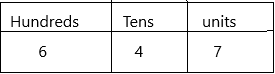6 is in the hundreds place
4 is in the tens place
7 is in the one’s place.
This can be written as in expanded form:
600+40+7=647
Question 2.
nine hundred twenty-one9 is in the hundreds place
2 is in the tens place
1 is in the one’s place.
This can be written as in expanded form:
900+20+1=921

Write the number using words.

Question 3.
562
Explanation: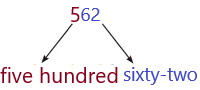Note: Learn to write numbers from 1 to 9. This is where it all starts. Learn these, and the rest gets much easier:
1. Understanding the place values: We can tell what each digit in a number means based on where it is.
2. Write the last two digits at the end of the number. This part is just like writing a normal two-digit number.
– 562: Start with just the 62 at the end. Write this as sixty-two
3. Write the hundreds place. The next digit is the hundreds place. This is an easy one. Just write the number in that place, then add the word “hundred.” Write this in front of your number.
– In 506, the 5 is in the hundreds place. Write this as five hundred.
4. Now we have five hundred sixty-two.

Question 4.
Draw and label points to show where 25 and 61 belong. (TEKS 2.2.E)To make a number line or to plot a number on it, follow the steps given below:
1. Step 1: Draw a horizontal line with arrows on both ends. [You can also draw a vertical number line]
2. Step 2: Choose a scale depending on the given number. For example, if you have to plot 5, then you can choose a scale of 1. If you have to plot 50, you can choose a scale of either 5, 10, or 25. [Determining an appropriate scale depends on the factors of the given number]
3. Step 3: Mark the points at equal intervals. So, if you have chosen 10 as the scale, then mark 10, 20, 30, 40, and so on.
4. Step 4: Locate the given number. Highlight that point with a circle.

Question 5.
Write how many hundreds, tens, and ones are shown. Then use blocks. Draw and write another way to show the number. (TEKS 2.2.A)
123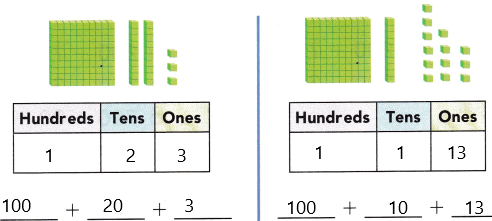Explanation:
First representation:
There is 1 block of hundreds which is equivalent to each block being 100.
There are 2 ten of blocks which are equivalent to each block is 10
There are 3 ones of block which is equivalent to each block is 1
The total marbles are 1*100=100
2*10=20
3*1=3
Therefore, the total marble is 100+20+3.
the total:123
Second representation:
There are 1 block of hundreds which is equivalent to each block being 100.
There are 1 ten of blocks which are equivalent to each block is 10
There are 13 ones of block which is equivalent to each block is 1
The total marbles are 1*100=100
1*10=10
13*1=13
Therefore, the total marble is 100+10+13
the total:123.

Fill in the bubble for the correct answer choice.

Question 6.
There are four hundred twelve pennies in a jar. Which shows this number?(A) 400
(B) 420
(C) 412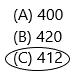Explanation:
The number of pennies in a jar=412.
The word form is given. Now we have to write in the number form.
4 is in the hundreds place
1 is in the tens place
2 is in the one’s place.

Question 7.
There are 10 crackers on each plate. There are 16 plates in all. How many crackers are there? (TEKS 2.2.A)(A) 160
(B) 116
(C) 206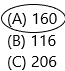Explanation:
The number of crackers in each plate=10
The number of crackers in all=16
The total number of crackers=16*10=160
Therefore, there are 160 crackers.

Question 8.
Ben draws a point on the number line. Which number belongs at the point? (TEKS 2.2.F)(A) 67
(B) 65
(C) 70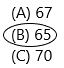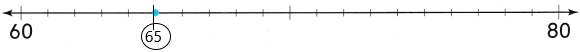To make a number line or to plot a number on it, follow the steps given below:
1. Step 1: Draw a horizontal line with arrows on both ends. [You can also draw a vertical number line]
2. Step 2: Choose a scale depending on the given number. For example, if you have to plot 5, then you can choose a scale of 1. If you have to plot 50, you can choose a scale of either 5, 10, or 25. [Determining an appropriate scale depends on the factors of the given number]
3. Step 3: Mark the points at equal intervals. So, if you have chosen 1 as the scale, then mark 60, 61, 62, 63, 64 and so on.
4. Step 4: Locate the given number. Highlight that point with a circle.

Question 9.
Tammy has 237 marbles. Which is another way to show this number? (TEKS 2.2.B)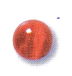(A) 200 + 70 + 3
(B) 200 + 30 + 7
(C) 700 + 30 + 2